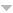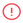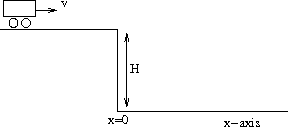# Documents

For page specific messages
For page specific messages
1927 records found.
Operations
Title & Summary Author Updated### $$\S\S$$ 3.6 :: Discontinuity across the branch cut

The solutions for probblems in chapter 3 appear here. To go to  desired section expand the Table of Contents by clicking there.

kapoor 01/03/19

### $$\S\S$$ 3.2 :: Tutorial ---The square root branch cutSummary (or abstract) not available for this node.
kapoor 01/03/19

### $$\S\S$$ 3.1 :: Questions ----------- Branch PointSummary (or abstract) not available for this node.
kapoor 01/03/19

### Is it difficult to share learning content?

No, if it is already published on Internet.

Yes, if it is mine and is in PDF/DOC/PPT format.

ranjan 01/03/19

kapoor 28/02/19

kapoor 28/02/19

kapoor 28/02/19

### QM-05031 :: Temperature of Sun

Consider an ionised plasma of protons in thermal equilibrium. Assuming a Maxwell-Boltzmann distribution, estimate the temperature required for two protons to overcome the Coulomb barrier for fusion assuming an approach distance of 1 fm. Compare this with the temperature when quantum effects come into play. For this, use the distance between the two protons as the de-Broglie wavelength at which Coulomb energy becomes equal to the kinetic energy of the protons.

See attached document for solution.

kapoor 28/02/19

### QM-13001 :: Car on a Ramp

 Consider a one dimensional motion of a particle along the x-axis under the action of a potential $V(x)=V_0>0$ for $x<=0$ and $V(x)=0$ for $x>0$. If the particle moves to the right from $x<0$, with an energy $2V_0$, standard quantum mechanics gives for the reflection coeffient at $x=0$ of the $0(1)$ ( The exact number is $(|(\sqrt{2}-1|/|\sqrt{2}+1|)^{1/2}$) and the result is independent of the mass of the particle. Now consider a car travelling with a speed $v$ towards a cliff ( height $H$) as shown in the figure. From the previous calculation the probability of reflection at $x=0$ should be $0(1)$ ( Assume the kinetic energy of the car to be of the same order as $mgH$ where $m$ is the mass of the car.). This is an absurd result.Do a correct modelling for the cliff and obtain a physically reasonable result.kapoor 28/02/19

### Problem/QFT-15005 Charged pion decay $$\pi^- \to e^- \bar{\nu}$$

The interaction Hamiltonian for pion decay $$\pi^-\longrightarrow e^- + \bar{\nu}$$ can be written as $H_\text{int} = \frac{g}{\sqrt{2}}\bar{\psi}_e(x)\gamma_\mu(1-\gamma_5)\psi(x)_\nu \partial^\mu \phi_\pi^-(x) + h.c.$

1. Show that the decay rate is given by $\Gamma = \frac{g^2}{8\pi} \frac{m_e^2(m_\pi^2-m_e^2)^2}{m_\pi^3}.$
2. Assuming that the coupling constant for $$\pi^-\longrightarrow \mu^- +\bar{\nu}$$ is equal to that for the electron decay, calculate the branching ratio $\frac{\Gamma(\pi^-\longrightarrow \mu^- +\bar{\nu})} {\Gamma((\pi^-\longrightarrow e^- + \bar{\nu})}$
kapoor 28/02/19